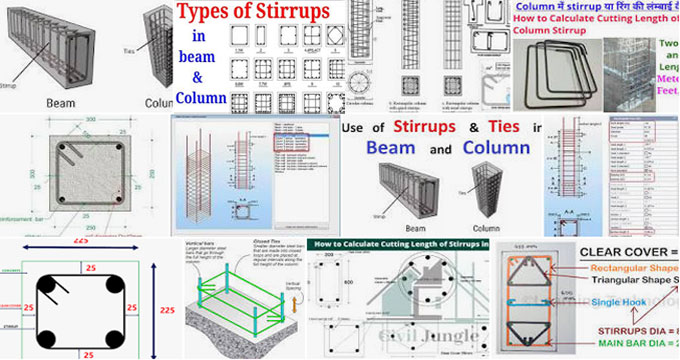# Making Process of Column StirrupThe reinforced concrete column is a structural member and is designed to bear loads. This column has two types of bar. One is a longitudinal bar and another one is a horizontal bar. Stirrups and ties are used in columns in the case of the horizontal bar.

Stirrups are also known as ring, shear reinforcement. Users have to calculate the cutting length of the bar and also have to calculate the number of stirrups to make stirrup for the column. After that users have to decide the size of the stirrup.

### Step by step guide

1. To determine the size of the stirrup.
2. To calculate the cutting bar length for stirrup.
3. To calculate the number of stirrups.
4. To bend the bar in stirrup?s shape.

### To determine the size of the stirrup

Let us say, The column size is 20" x 40". We all know that the concrete clear cover for reinforcement of column is 1??.

So the length of the stirrup will be 40"-2?1??=37" and the width will be 20"-2?1??=17".

So the size of the stirrup is 37"x17".

### To calculate Cutting-Bar-Length for Stirrup

Users should have to calculate the cutting bar length for the stirrup before cutting the bar. There are two types.

A. Actual bar length and
B. Cutting bar length.

Actual bar length of the stirrup is
2 x length + 2 x width + 2 x hook?s length= 2 x 37" + 2 x 17" + 2 x 3" = 114" or 9.5'.

Hook length for 10mm? bar is 6 x 10 = 60 mm.

Here, the hook length is calculated by a formula that is 6db (diameter of the bar).

According to the code length of the hook should not be less than 75mm.

So the hook length for the stirrup is 3" (75mm = 3").

The actual bar length of the stirrup is 9.5'.

Here the cutting bar length will be less than the actual bar length. The stirrup has three 90? bend and two 135? hooks. For each and every bend, the length of the bar will be reduced. There is a certain formula to calculate the reduced length for the bend.

Below a chart is given to reduced length for a 90? bend for different diameters of the bar.

10 mm? bar ? 1" 12 mm? bar ? 1?? 16 mm? bar ? 1??

A 10 mm? bar for our stirrup so the cutting bar length will be

9.5' ? 3 x 1" (bend deduction is ignored for 135? bend). = 9'-2"

So, users have to cut the 9'-2" length of 10mm? bar. That means, cutting bar length for the stirrup is 9'-2".

### To calculate the Number of Stirrups

Stirrups have two types.

1. S1. S1 is spaced 4" c/cS2 is spaced 8" c/c.
2. S2. S2 is spaced 8" c/c

S1 is provided in the bottom ?th portion and top ?th portion of the length of the clear column. S2 is provided in the middle half portion of the length of length.

Here the length of a clear column means the height of the column between the top of the floor to the bottom of the beam.

### Calculator to get the numbers of stirrups for the column

The floor height is 10'. Beam height is 2

Clear height of the column is = floor height ? beam height = 10' ? 2' =8'

Number of stirrups are = 4?/4" + 4?/8" + 2 + 1 = 21 nos.

(1 bar should be added to get the correct number of stirrups.)

### To bend the bar in stirrup?s shape

Users can use stirrup-making-machine to complete large projects where a huge quantity of stirrups is required. Users are also able to make the stirrups by hand in the projects. To complete the process users have to need a stand and a handle.

Users have to place the rod in the stand and bend the rod with the handle. After that users have to go for the trial and error method to get the desired size of the stirrup. Users should not forget to make the hook angle at 135?.

? ? inch.
? ? foot.
? ? degree.
DB ? diameter of the bar.
nos ? numbers.
? ? diameter.
c/c ? center to center distance.